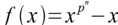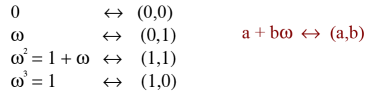Courses

# Finite Fields - Field Theory Mathematics Notes | EduRev

## Algebra for IIT JAM Mathematics

Created by: Veda Institute

## Mathematics : Finite Fields - Field Theory Mathematics Notes | EduRev

The document Finite Fields - Field Theory Mathematics Notes | EduRev is a part of the Mathematics Course Algebra for IIT JAM Mathematics.
All you need of Mathematics at this link: Mathematics

Basic Definitions

A field is a set F with two binary operations + and × such that:

1) (F, +) is a commutative group with identity element 0.
2) (F-{0},×) is a commutative group with identity element 1.
3) The distributive law a(b+c) = ab + ac holds ∀ a,b,c ∈ F.

Examples: ℝ, ℚ, ℂ, ℤp for p a prime are fields with the usual operations of addition and multiplication.

subfield of a field F is a subset of F which is itself a field with the same operations as F.

Examples: ℚ is a subfield of ℝ. ℝ is a subfield of ℂ. ℤp has no subfields (other than itself)

Characteristic of a Field

Since 1 is in any field and addition is a closed operation (the sum of any two elements is another element of the field) we have that; 1, 1+1, 1+1+1, 1+1+1+1, 1+1+1+1+1, etc. are all elements of the field. Two possibilities exist for this sequence of elements – either some sum of 1's will equal 0 (in which case the sequence cycles through some finite set of values) or not (in which case none of the elements of the sequence are the same and we get an infinite number of elements in the field).

The smallest positive number of 1's whose sum is 0 is called the characteristic of the field. If no number of 1's sum to 0, we say that the field has characteristic zero.

Prime Subfield

It can be shown (not difficult) that the characteristic of a field is either 0 or a prime number.

If the characteristic of a field is p, then the elements which can be written as sums of 1's form a ℤp inside the field, i.e., a subfield.

This subfield is the smallest subfield that the field can contain.

If the characteristic of the field is 0, then these elements form a copy of the natural numbers inside the field. This set of elements together with their additive and multiplicative inverses create a copy of ℚ, the rational numbers, inside the field. Again, this must be the smallest subfield contained in the field.
The smallest subfield of a field is called the prime subfield and it is either a ℤp or ℚ.

Extension Fields

If K is a subfield of a field L, then we say that L is an extension (or extension field) of K. Every field is thus an extension of its prime subfield.

A field may always be viewed as a vector space over any of its subfields. (The field elements are the vectors and the subfield elements are the scalars). If this vector space is finite dimensional, the dimension of the vector space is called the degree of the field over its subfield. A finite field must be a finite dimensional vector space, so all finite fields have degrees.

The number of elements in a finite field is the order of that field.

The order of a finite field

A finite field, since it cannot contain ℚ, must have a prime subfield of the form GF(p) for some prime p, also:

Theorem - Any finite field with characteristic p has pn elements for some positive integer n. (The order of the field is pn.)

Proof: Let L be the finite field and K the prime subfield of L. The vector space of L over K is of some finite dimension, say n, and there exists a basis α12, ... ,αn of L over K. Since every element of L can be expressed uniquely as a linear combination of the αi over K, i.e., every a in L can be written as, a = ∑βiαi , with βi in K, and since K has p elements, L must have pn elements.

Splitting Fields

The previous result does not prove the existence of finite fields of these sizes. To prove existence we need to talk about polynomials.

Given a polynomial with coefficients in a field K, the smallest extension of K in which the polynomial can be completely factored into linear factors is called a splitting field for the polynomial.

Ex: The polynomial x2 + 1 does not factor over ℝ, but over the extension ℂ of the reals, it does, i.e., x2 + 1 = (x + i)(x – i). Thus, ℂ is a splitting field for x2 + 1.

Theorem: If f(x) is an irreducible polynomial with coefficients in the field K, then a splitting field for f(x) exists and any two such are isomorphic.

Finite Fields

Theorem: The splitting field ofthought of as a polynomial over GF(p) has pn elements, and is denoted GF(pn).

Corollary: For each prime p and positive integer n, the field GF (pn) exists and is unique (two fields of the same order are isomorphic).

Recall that we have already mentioned that GF(pn) – {0} = GF(pn)* is a cyclic group under multiplication, and the generators of this group are called primitive elements of the field.

Constructing Finite Fields

There are several ways to represent the elements of a finite field. The text describes a representation using polynomials. This method is a bit cumbersome for doing calculations. We will give other representations that are more computationally friendly.

Using the fact that a field is a vector space over its prime subfield it is easy to write all the elements as vectors.

Example: GF(4) is a 2-dimensional vector space over GF(2), so its four elements can be written as (0,0), (0,1), (1,0) and (1,1). Adding these elements is done componentwise (in GF(2)).

Multiplication however is more complicated and involves a strange rule ... so this is not a great way to represent the field.

Constructing Finite Fields

Another idea that can be used as a basis for a representation is the fact that the non-zero elements of a finite field can all be written as powers of a primitive element.

Example: Let ω be a primitive element of GF(4). The elements of GF(4) are then 0, ω, ω2, ω3. Multiplication is easily done in this representation (just add exponents mod 3), but addition is not obvious.

If we can link these two representations together, we will easily be able to do both addition and multiplication.

Example: In GF(4) we have:Constructing Finite Fields

The task is thus to locate a primitive element and set up this table of correspondences.

In GF(pn) with n > 1, a primitive element can not be in the prime subfield. Thus, we must seek them amongst the roots of irreducible polynomials over GF(p). In particular, they will be found as roots of irreducible polynomials of degree n, in fact, roots of primitive polynomials of degree n.

While we could determine whether or not an irreducible polynomial is primitive, it is often easier just to look at the roots of irreducible polynomials and see if they are generators. Also, we need only examine monic (leading coefficient is 1) polynomials since multiplying a polynomial by a non-zero scalar does not change its roots.

Offer running on EduRev: Apply code STAYHOME200 to get INR 200 off on our premium plan EduRev Infinity!

58 docs

,

,

,

,

,

,

,

,

,

,

,

,

,

,

,

,

,

,

,

,

,

;